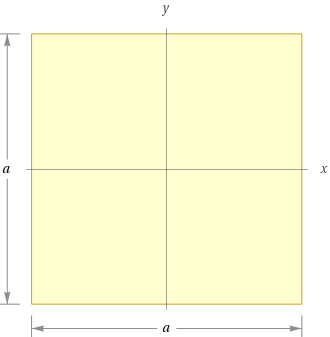Search IntMath
Close

# Understanding the Differences Between a Square and Rhombus In Geometry## What is a Square?

A square is a four-sided shape with four equal sides and four right angles. It is a type of rectangle, but it is the only rectangle where all four sides are equal and all four angles are equal. The opposite sides of a square are parallel, and the diagonals are equal. All of these characteristics make a square a very special type of figure.

## What is a Rhombus?

A rhombus is also a four-sided shape with four equal sides, but unlike a square, it has no right angles. The opposite sides of a rhombus are parallel, and the diagonals are equal. However, the angles of a rhombus are all different, usually ranging from 60 to 120 degrees.

## Comparison of a Square and a Rhombus

A square and a rhombus are both four-sided shapes with four equal sides. However, the major difference between these two shapes is that a square has four right angles and all of its angles are equal, while a rhombus has no right angles and its angles are not equal. Additionally, both shapes have parallel opposite sides and equal diagonals.

## Practice Problems

1. What type of shape has four equal sides and four right angles?

2. What type of shape has four equal sides and no right angles?

3. What is the difference between a square and a rhombus?
Answer: The major difference between a square and a rhombus is that a square has four right angles and all of its angles are equal, while a rhombus has no right angles and its angles are not equal.

4. Which of the following shapes has four equal sides and no right angles?

5. What is true of both a square and rhombus?
Answer: Both a square and a rhombus have parallel opposite sides and equal diagonals.

6. What is the sum of the angles in a square?
Answer: The sum of the angles in a square is 360 degrees.

7. What is the sum of the angles in a rhombus?
Answer: The sum of the angles in a rhombus is 360 degrees.

## Summary

This article explored the differences between a square and a rhombus in geometry. A square is a four-sided shape with four equal sides and four right angles, while a rhombus is also a four-sided shape with four equal sides but no right angles. The major difference between these two shapes is that a square has four right angles and all of its angles are equal, while a rhombus has no right angles and its angles are not equal. Additionally, both shapes have parallel opposite sides and equal diagonals.

## FAQ

### What is the difference between a square and a rhombus?

The main difference between a square and a rhombus is that a square has four equal sides and four equal angles, whereas a rhombus does not have four equal sides or four equal angles. The sides of a rhombus are all equal in length, but the angles are not. The angles of a square are all 90 degrees, while the angles of a rhombus can range from 60 to 120 degrees.

### What is the interior angle of a square?

The interior angles of a square are all 90 degrees.

## Problem SolverThis tool combines the power of mathematical computation engine that excels at solving mathematical formulas with the power of GPT large language models to parse and generate natural language. This creates math problem solver thats more accurate than ChatGPT, more flexible than a calculator, and faster answers than a human tutor. Learn More.